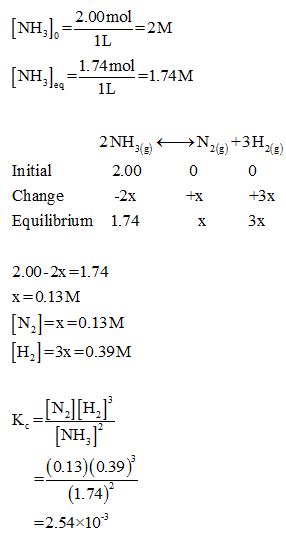# Ammonia is among the top ten synthesized compounds. Its many uses include the manufacture of ammonium nitrate and other fertilizers. Ammonia decomposes at high temperatures. In an experiment to explore this behavior, 2.00 moles of gaseous NH3 are sealed in a rigid 1-liter vessel. The vessel is heated at 800 K and some of the NH3 decomposes in the following reaction:2NH3​(g)<-->N2​(g)+3H2​(g) The system eventually reaches equilibrium and is found to contain 1.74 mole of NH3. What is the value of KC for the decomposition reaction at 800 K?What is the value of KP for the decomposition reaction at 800 K?

Question
169 views
Ammonia is among the top ten synthesized compounds. Its many uses include the manufacture of ammonium nitrate and other fertilizers. Ammonia decomposes at high temperatures. In an experiment to explore this behavior, 2.00 moles of gaseous NH3 are sealed in a rigid 1-liter vessel. The vessel is heated at 800 K and some of the NH3 decomposes in the following reaction:
2NH3​(g)<-->N2​(g)+3H2​(g)

The system eventually reaches equilibrium and is found to contain 1.74 mole of NH3. What is the value of KC for the decomposition reaction at 800 K?

What is the value of KP for the decomposition reaction at 800 K?

check_circle

Step 1

Given,

Initial moles of ammonia = 2.00mol.

Volume of vessel = 1L.

Equilibrium moles of ammonia = 1.74mol.

Step 2

The equilibrium constant Kc for the decomposition of ammonia can be calculated as,...

### Want to see the full answer?

See Solution

#### Want to see this answer and more?

Solutions are written by subject experts who are available 24/7. Questions are typically answered within 1 hour.*

See Solution
*Response times may vary by subject and question.
Tagged in